Number Puzzle - 1

# Number Puzzle - 1

Test Description

## 15 Questions MCQ Test Learn with Fun: Test Your Knowledge | Number Puzzle - 1

Number Puzzle - 1 for Class 5 2023 is part of Learn with Fun: Test Your Knowledge preparation. The Number Puzzle - 1 questions and answers have been prepared according to the Class 5 exam syllabus.The Number Puzzle - 1 MCQs are made for Class 5 2023 Exam. Find important definitions, questions, notes, meanings, examples, exercises, MCQs and online tests for Number Puzzle - 1 below.
Solutions of Number Puzzle - 1 questions in English are available as part of our Learn with Fun: Test Your Knowledge for Class 5 & Number Puzzle - 1 solutions in Hindi for Learn with Fun: Test Your Knowledge course. Download more important topics, notes, lectures and mock test series for Class 5 Exam by signing up for free. Attempt Number Puzzle - 1 | 15 questions in 15 minutes | Mock test for Class 5 preparation | Free important questions MCQ to study Learn with Fun: Test Your Knowledge for Class 5 Exam | Download free PDF with solutions
 1 Crore+ students have signed up on EduRev. Have you?
Number Puzzle - 1 - Question 1

### What number replaces the Question mark ?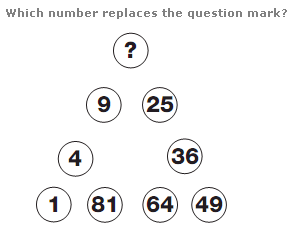Number Puzzle - 1 - Question 2

### What number replaces the Question mark ?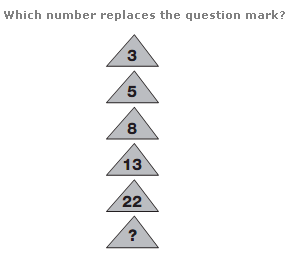Number Puzzle - 1 - Question 3

### What number replaces the Question mark ?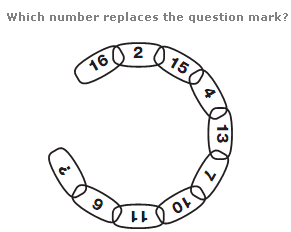Number Puzzle - 1 - Question 4
What number replaces the Question mark ?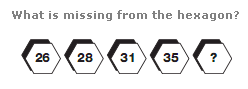Number Puzzle - 1 - Question 5
What number replaces the Question mark ?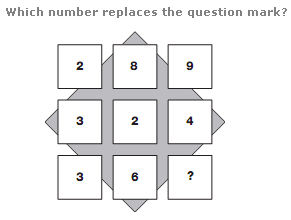Number Puzzle - 1 - Question 6
What number replaces the Question mark ?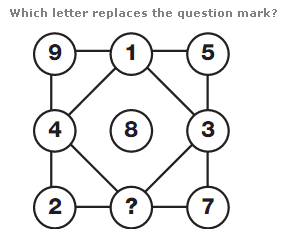Number Puzzle - 1 - Question 7
What number replaces the Question mark ?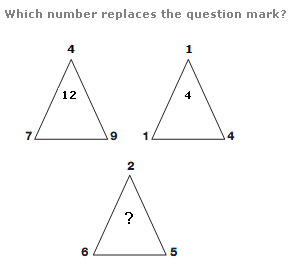Number Puzzle - 1 - Question 8
What number replaces the Question mark ?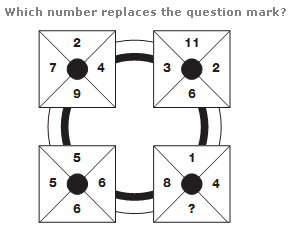Number Puzzle - 1 - Question 9
What number replaces the Question mark ?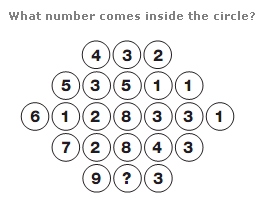Number Puzzle - 1 - Question 10
What number replaces the Question mark ?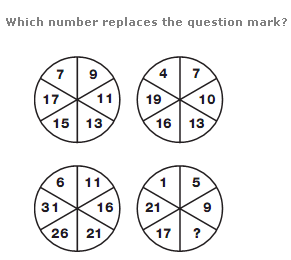Number Puzzle - 1 - Question 11
What number replaces the Question mark ?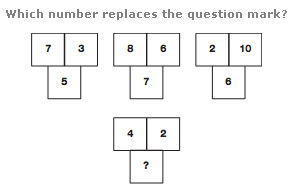Number Puzzle - 1 - Question 12
What number replaces the Question mark ?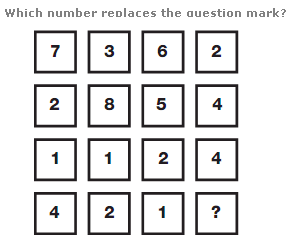Number Puzzle - 1 - Question 13
What number replaces the Question mark ?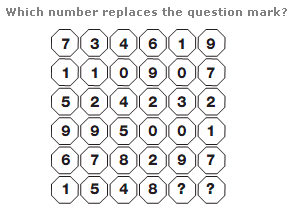Number Puzzle - 1 - Question 14
What number replaces the Question mark ?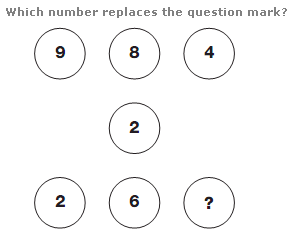Number Puzzle - 1 - Question 15
What number replaces the Question mark ?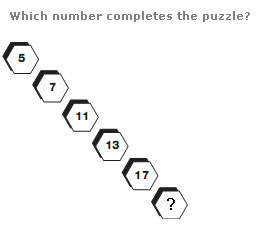## Learn with Fun: Test Your Knowledge

200 tests
 Use Code STAYHOME200 and get INR 200 additional OFF Use Coupon Code
Information about Number Puzzle - 1 Page
In this test you can find the Exam questions for Number Puzzle - 1 solved & explained in the simplest way possible. Besides giving Questions and answers for Number Puzzle - 1, EduRev gives you an ample number of Online tests for practice

200 tests